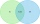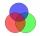# Subtracting + intersection of sets - math problems

#### Number of problems found: 6

• Subtracting setsFor two sets K, L is true: K has 30 elements, L has 27 elements and the set L - K has 22 elements. How many elements does the set K - L have?
• Ten pupils10 pupils came to the art group. 8 pupils painted with watercolors and 9 pupils painted with ink, each pupil painted with ink or watercolors. How many pupils painted water and ink at the same time?
• Intersect and conjuctionLet U={1,2,3,4,5,6} A={1,3,5} B={2,4,6} C={3,6} Find the following. 1. )AUB 2. )A'UB'
• Square and circlesThe square in the picture has a side length of a = 20 cm. Circular arcs have centers at the vertices of the square. Calculate the areas of the colored unit. Express area using side a.
• Cutting the prismA prism with a square base with a content of 1 cm2 and a height of 3 cm was cut from a cube with an edge length of 3 cm. What is the surface of the body formed from the cube after cutting the prism?
• The HotelThe Holiday Hotel has the same number of rooms on each floor. Rooms are numbered with natural numerals sequentially from the first floor, no number is omitted, and each room has a different number. Three tourists arrived at the hotel. The first one was in

We apologize, but in this category are not a lot of examples.
Do you have an interesting mathematical word problem that you can't solve it? Submit a math problem, and we can try to solve it.

We will send a solution to your e-mail address. Solved examples are also published here. Please enter the e-mail correctly and check whether you don't have a full mailbox.

Please do not submit problems from current active competitions such as Mathematical Olympiad, correspondence seminars etc...

Subtracting Problems. Intersection of sets - math word problems.